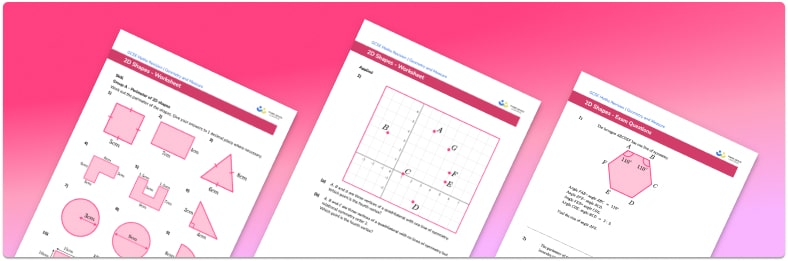# Area Of 2D Shapes Worksheet• Section 1 of the 2D shapes worksheet contains 20+ skills-based 2D shapes questions, in 3 groups to support differentiation
• Section 2 contains 3 applied 2D shapes questions with a mix of worded problems and deeper problem solving questions
• Section 3 contains 3 foundation and higher level GCSE exam style 2D shapes questions
• Answers and a mark scheme for all 2D shapes questions are provided
• Questions follow variation theory with plenty of opportunities for students to work independently at their own level
• All questions created by fully qualified expert secondary maths teachers
• Suitable for GCSE maths revision for AQA, OCR and Edexcel exam boards

• This field is for validation purposes and should be left unchanged.

You can unsubscribe at any time (each email we send will contain an easy way to unsubscribe). To find out more about how we use your data, see our privacy policy.

### 2D shapes at a glance

A 2D shape is flat and has two dimensions – for example, length and width. At GCSE, the 2D shapes studied include: triangles, quadrilaterals and other higher-order polygons (n- number of sides), as well as circles and fractions of circles.

Area is the amount of space covered by a 2D shape; the metric units for area are square units such as square centimetres (cm2), square metres (m2) and square millimetres (mm2). Area of 2D shapes is a key topic at GCSE, including the area of a rectangle, the area of a triangle, and the area of quadrilaterals such as the area of a parallelogram and area of a trapezium. The area of compound shapes (shapes made up of two or more simple shapes) is calculated by summing the area of two or more shapes, or subtracting a component from another 2D shape.

Students are also expected to be able to find the area of a circle and fractions of a circle, extending to finding the area of a sector. It is important to be confident working with decimals and fractions for this topic, in addition to integer lengths and dimensions.

Calculating area is an important skill for application to 3D shape work, including finding the surface area of a 3D shape, and the area of a cross section when calculating the volume of prisms.

Angle properties, particularly in polygons, is another 2D shapes key topic. A regular polygon has equal side lengths and equal angles. A square is a regular polygon because each side length is equal and each interior angle is equal, whereas a rhombus is not regular because although a rhombus has equal sides, it does not have equal angles.

2D shapes are rectilinear if all of the side lengths are at 90 degrees (right angles) to one another, such as a square or a rectangle. The further study of angles and properties of triangles is called trigonometry.

The topic 2D shapes also includes symmetry, identifying lines of symmetry and rotational symmetry in various polygons.

Looking forward, students can then progress to additional geometry worksheets, for example the quadrilaterals worksheet, the construction of triangles worksheet, or the vertices, edges and faces worksheet.For more teaching and learning support on Geometry our GCSE maths lessons provide step by step support for all GCSE maths concepts.

## Do you have KS4 students who need more focused attention to succeed at GCSE?There will be students in your class who require individual attention to help them succeed in their maths GCSEs. In a class of 30, it’s not always easy to provide.

Help your students feel confident with exam-style questions and the strategies they’ll need to answer them correctly with our dedicated GCSE maths revision programme.

Lessons are selected to provide support where each student needs it most, and specially-trained GCSE maths tutors adapt the pitch and pace of each lesson. This ensures a personalised revision programme that raises grades and boosts confidence.

Find out more